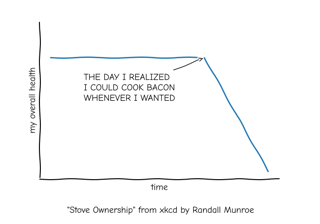# matplotlib.pyplot.xkcd#

matplotlib.pyplot.xkcd(scale=1, length=100, randomness=2)[source]#

Turn on xkcd sketch-style drawing mode. This will only have effect on things drawn after this function is called.

For best results, the "Humor Sans" font should be installed: it is not included with Matplotlib.

Parameters:
scalefloat, optional

The amplitude of the wiggle perpendicular to the source line.

lengthfloat, optional

The length of the wiggle along the line.

randomnessfloat, optional

The scale factor by which the length is shrunken or expanded.

Notes

This function works by a number of rcParams, so it will probably override others you have set before.

If you want the effects of this function to be temporary, it can be used as a context manager, for example:

```with plt.xkcd():
# This figure will be in XKCD-style
fig1 = plt.figure()
# ...

# This figure will be in regular style
fig2 = plt.figure()
```

## Examples using `matplotlib.pyplot.xkcd`#XKCD

XKCD